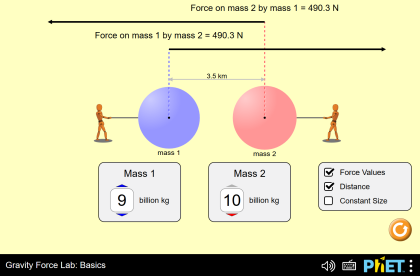All the community
search

# Gravity Force Lab: Basics

## Descripción

Visualize the gravitational force that two objects exert on each other. Discover the factors that affect gravitational attraction, and determine how adjusting these factors will change the gravitational force.

Objetivos de Aprendizaje

• Relate the gravitational force to masses of objects and distance between objects
• Explain Newton's third law for gravitational forces
• Use measurements to determine the universal gravitational constant• Actions more_vert

Author